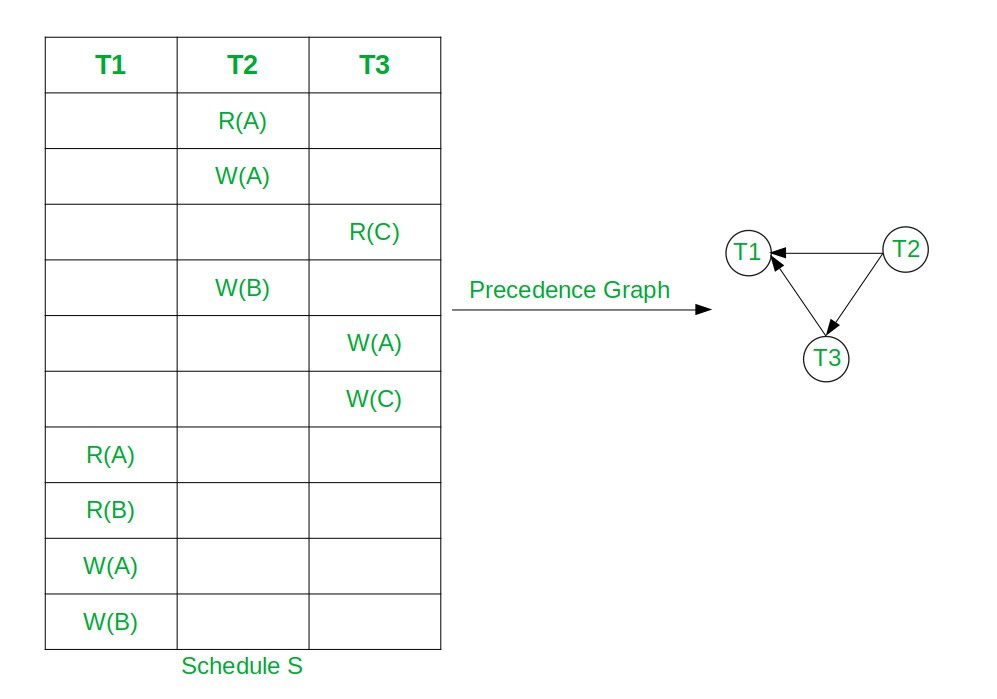# Equivalent Serial Schedule of Conflict Serializable Schedule in DBMS

• Difficulty Level : Hard
• Last Updated : 30 Sep, 2021

Prerequisite: Conflict Serializability, Precedence Graph

Conflict Serializable Schedule: A schedule is called conflict serializable if it can be transformed into a serial schedule by swapping non-conflicting operations. The serial schedule of the Conflict Serializable Schedule can be found by applying topological Sorting on the Precedence Graph of the Conflict Serializable Schedule.

Note: Precedence Graph of Conflict Serial Schedule is always directed acyclic graph.

Approach: Follow to below steps to find topological sorting of Precedence Graph:

• Find the indegree of all nodes for the given Precedence Graph and store it in an auxiliary array.
• Now For each node having indegree 0 perform the following:
• Print the current node T as the order of the topological sort.
• Let the node T be the node with in-degree 0.
• Remove T and all edges connecting to T from the graph.
• Update indegree of all nodes after the above steps.
• After the above steps, the topological sort of the given precedence graph can be calculated.

Below is the illustration of the above approach:

Let, the Conflict Serial Schedule be S: R2(A) W2(A) R3(C) W2(B) W3(A) W3(C) R1(A) R2(B) W1(A) W2(B)• Here node T2 has indegree 0.
• So, select T2 and remove T2 and all edges connecting from it.
• Now T3 has indegree 0. So, select T3 and remove the edge T3→T1.
• At the end select T3. So the topological Sorting is T2, T3, T1.
• Hence, the equivalent serial schedule of given conflict serializable schedule is T2→T3→T1, i.e., S2: R2(A) W2(A) W2(B) R3(C) W3(A) W3(C) R1(A) R2(B) W1(A) W1(B).

There may be more than one equivalent serial schedule of the conflict serializable schedule.

Below is the implementation to get the Serial Schedule of CSS using Topological Sorting:

## C++

 `// C++ program to print serial schedule``// of CSS using Topological sorting``#include ``using` `namespace` `std;` `class` `PrecedenceGraph {` `    ``// No. of vertices``    ``int` `V;` `    ``// Pointer to an array containing``    ``// adjacency vector``    ``vector<``int``>* adj;` `    ``// Vector to store SerialSchedule``    ``vector<``int``> serialSchedule;` `    ``// Function  declarations``    ``void` `computeIndegree(``int``* indegree);``    ``int` `findZeroIndegree(``int``* indegree,``                         ``bool``* visited);` `public``:``    ``// Constructor``    ``PrecedenceGraph(``int` `V);` `    ``// Function declarations``    ``void` `addEdge(``int` `v, ``int` `w);``    ``void` `topologicalSort();``    ``void` `printSchedule();``};` `// Function to create the precedence``// graph``PrecedenceGraph::PrecedenceGraph(``int` `V)``{``    ``this``->V = V;``    ``adj = ``new` `vector<``int``>[V];``}` `// Function to add the edge to the``// precedence graph``void` `PrecedenceGraph::addEdge(``int` `v,``                              ``int` `w)``{``    ``adj[v].push_back(w);``}` `// Function to compute indegree of nodes``void` `PrecedenceGraph::computeIndegree(``    ``int``* indegree)``{``    ``for` `(``int` `i = 1; i < V; i++) {` `        ``// Traverse the adjacency list``        ``// of node i``        ``for` `(``int` `j = 0;``             ``j < adj[i].size(); j++) {``            ``int` `node = adj[i][j];` `            ``// Update the indegree``            ``// of node``            ``indegree[node]++;``        ``}``    ``}``}` `// Function to find node with``// zero indegree``int` `PrecedenceGraph::findZeroIndegree(``    ``int``* indegree, ``bool``* visited)``{``    ``for` `(``int` `i = 1; i < V; i++) {` `        ``// If node is not visited``        ``// and have indegree 0``        ``if` `(!visited[i]``            ``&& indegree[i] == 0) {` `            ``// Mark node visited``            ``visited[i] = ``true``;` `            ``// Return node``            ``return` `i;``        ``}``    ``}` `    ``// All nodes are visited``    ``return` `-1;``}` `// Function to find the topological``// Sorting of the given graph``void` `PrecedenceGraph::topologicalSort()``{``    ``// Create and initialize``    ``// visited array``    ``bool``* visited = ``new` `bool``[V]();` `    ``// Create and initialize``    ``// indegree array``    ``int``* indegree = ``new` `int``[V]();` `    ``computeIndegree(indegree);` `    ``// Check if the node with``    ``// indegree 0 is available``    ``int` `node = findZeroIndegree(``        ``indegree, visited);` `    ``bool` `nodeAvailable = ``false``;` `    ``if` `(node != -1) {``        ``nodeAvailable = ``true``;``    ``}``    ``while` `(nodeAvailable) {` `        ``// Add node to serial schedule``        ``serialSchedule.push_back(node);` `        ``for` `(``int` `i = 0;``             ``i < adj[node].size(); i++) {` `            ``// Delete all edges of current``            ``// node and update indegree``            ``indegree[adj[node][i]]--;``        ``}` `        ``// Find next node with indegree 0``        ``node = findZeroIndegree(indegree,``                                ``visited);` `        ``if` `(node == -1) {` `            ``// Node with zero indegree``            ``// not available``            ``nodeAvailable = ``false``;``        ``}``    ``}``}` `// Function to print the serial schedule``void` `PrecedenceGraph::printSchedule()``{``    ``for` `(``int` `i : serialSchedule) {``        ``cout << ``"T"` `<< i << ``" "``;``    ``}``}` `// Driver Code``int` `main()``{``    ``// Create a precedence graph``    ``// given in the above diagram``    ``PrecedenceGraph graph(4);``    ``graph.addEdge(2, 1);``    ``graph.addEdge(2, 3);``    ``graph.addEdge(3, 1);` `    ``// Find topological ordereing``    ``graph.topologicalSort();` `    ``// Print Schedule``    ``cout << ``"Equivalent Serial"``         ``<< ``" Schedule is :"``;``    ``graph.printSchedule();``}`

Output:

`Equivalent Serial Schedule is :T2 T3 T1`

Time Complexity: O(N)
Auxiliary Space: O(N)

My Personal Notes arrow_drop_up